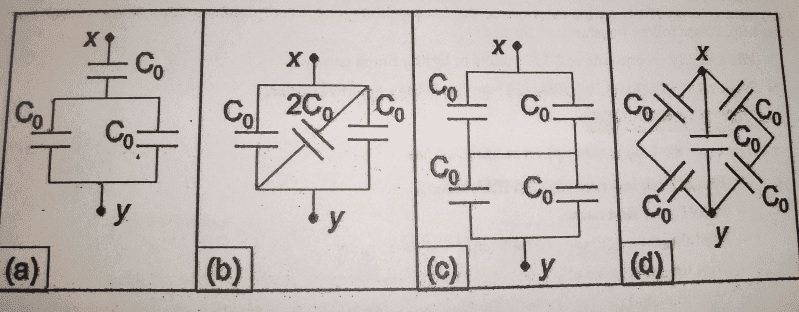# Determining Capacitance in Different Kinds of Capacitors

## Homework Statement

Determine the equivalent capacitance (in terms of C0) between points x and y of each network of capacitors shown.## Homework Equations

For capacitors connected in parallel, Ceq=C1+C2+.....Cn

For capacitors connected in series,
Ceq=1/C1+1/C2+.....1/Cn

## The Attempt at a Solution

This is my first time learning about capacitors, and I am just learning about the difference between series and parallel capacitors. Sometimes it's hard for me to tell the difference, especially with some of the different geometries that are given. I know that in parallel capacitors, capacitors are connecting with equal voltage drops across the capacitors, and in series capacitors, capacitors are connected with equal charges on the capacitors. First, I think I should combine the capacitors in parallel to get Ceq', then combine the remaining capacitors in series into one final Ceq.

a)So, to take this slowly, with part a, the two capacitors that are opposite to each other are parallel. So Ceq'=2C0 Now to combine it with the remaining capacitor in series, Ceq=1/2C0 + 1/C0 = 3/2C0

b) I'm not sure what to do when they are diagonal to each other. Are they series?

c) In the top box there is 2C0 in the bottom box there is 2C0, since they are in series, Ceq=1/2C0+1/2C0=1/C0

d) Same problem with B.

sweetdion

rl.bhat
Homework Helper
a) Ceq=1/2C0 + 1/C0 = 3/2C0
It should be
1/Ceq=1/2C0 + 1/C0 = 3/2C0
b)Al the three are in parallel, one plate of each capacitor is connected to x and the other to y.
c) 1/ceq = 1/co
d) Left and right branches are in series, the the parallel combination.

b) Ceq=2C0+C0+C0=4C0
d) 1/Ceqleft=1/C0+1/C0 ---> Ceqleft = C0/2 = Ceqright
Ceqtot=C0/2 + C0/2 + C0 --->2C0
correct?

rl.bhat
Homework Helper
Correct.

Thanks alot for your help!